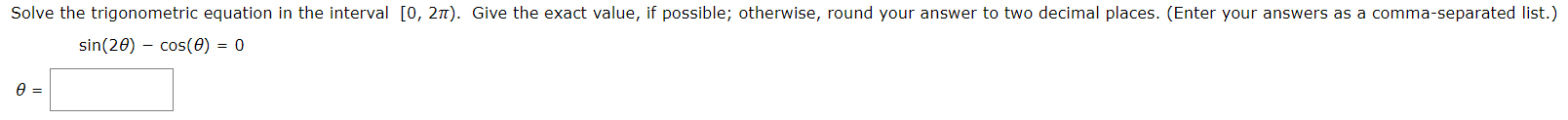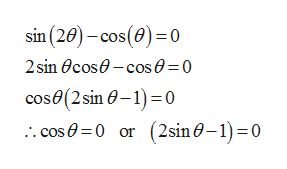# Solve the trigonometric equation in the interval [0, 27). Give the exact value, if possible; otherwise, round your answer to two decimal places. (Enter your answers as a comma-separated list.)sin(20) cos(0) = 0

Question
8 viewshelp_outlineImage TranscriptioncloseSolve the trigonometric equation in the interval [0, 27). Give the exact value, if possible; otherwise, round your answer to two decimal places. (Enter your answers as a comma-separated list.) sin(20) cos(0) = 0 fullscreen
check_circle

Step 1

The given trigonometric equation is,

Step 2

Solve the trigonometric equation as follows.help_outlineImage Transcriptionclosesin (20)-cos (0)0 2sin Ocos-cos 0=0 cose(2sin 0-1 0 .cos 0 0 or (2sin0-1) 0 fullscreen
Step 3

The solution when the cosine term is equa...

### Want to see the full answer?

See Solution

#### Want to see this answer and more?

Solutions are written by subject experts who are available 24/7. Questions are typically answered within 1 hour.*

See Solution
*Response times may vary by subject and question.
Tagged in

### Calculus